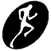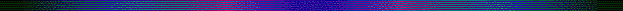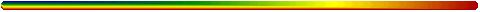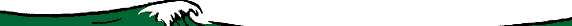TerminologyMeter is the standard international measure since 1791 based on the decimal system.

The original terminology came from the French National Assembly as a suggestion for the reform of the complicated national system of weights and measures by the Paris Academy of Sciences. In the intervening years, the exact length of the meter was refined.

Measure it?

1-D

3.2808399 feet equal a meter.

so 1898 X 3.2808399 = 6227.04 feet.2-D

87120 square feet in two acres means that

an acre = 43560 square feet.

an acre is also .404685642 hectares3-D

one cubic centimeter of water is equal [=] to one gram of water.4-D

99 people per day die in automobile accidents for over sixty years.

nearly 36,500 die annually = 2,190,000 deaths in US since 1962.Parts of a meter?

What is a metric?

Millimeter (mm)
Length unit equal to 1 x 10-3 meter or 0.001 meter.

Micron (µm)
Length unit equal to 1 x 10-6 meter or 0.000001 meter.

Nanometer (nm)
Length unit equal to 1 x 10-9 meter or 0.000000001 meter.

Angstrom Unit
Length unit equal to 1 x 10-10 meter or 0.0000000001 meter, or 1 x 10-8 centimeter. It is used for atomic measurements and radiation wavelengths..prefix symbol exponent number fraction terms
centi
c
10-2
0.01
hundredth
milli
m
10-3
0.001
thousandth
micro
μ
10-6
0.000 001
millionth
nano
n
10-9
0.000 000 001
billionth